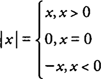## Absolute Value

The concept of absolute value has many applications in the study of calculus. The absolute value of a number x, written | x| may be defined in a variety of ways. On a real number line, the absolute value of a number is the distance, disregarding direction, that the number is from zero. This definition establishes the fact that the absolute value of a number must always be nonnegative—that is, | x|≥0.

A common algebraic definition of absolute value is often stated in three parts, as follows:Another definition that is sometimes applied to calculus problems isor the principal square root of x 2. Each of these definitions also implies that the absolute value of a number must be a nonnegative.